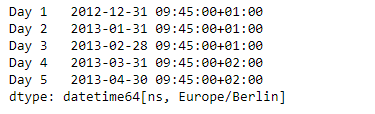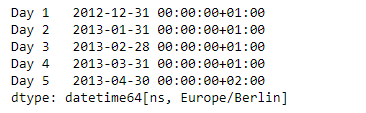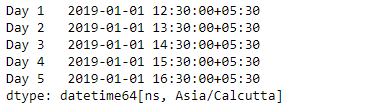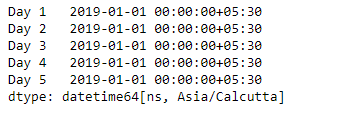# Python | Pandas Series.dt.normalize

`Series.dt` can be used to access the values of the series as datetimelike and return several properties. Pandas` Series.dt.normalize()` function convert times to midnight. The time component of the date-time is converted to midnight i.e. 00:00:00. This is useful in cases, when the time does not matter. Length is unaltered. The timezones are unaffected.

Syntax: Series.dt.normalize(*args, **kwargs)

Parameter : None

Returns : DatetimeArray, DatetimeIndex or Series

Example #1: Use `Series.dt.normalize()` function to convert the times in the given series object to midnight.

 `# importing pandas as pd ` `import` `pandas as pd ` ` `  `# Creating the Series ` `sr ``=` `pd.Series(pd.date_range(``'2012-12-31 09:45'``, periods ``=` `5``, freq ``=` `'M'``, ` `                            ``tz ``=` `'Europe / Berlin'``)) ` ` `  `# Creating the index ` `idx ``=` `[``'Day 1'``, ``'Day 2'``, ``'Day 3'``, ``'Day 4'``, ``'Day 5'``] ` ` `  `# set the index ` `sr.index ``=` `idx ` ` `  `# Print the series ` `print``(sr) `

Output :Now we will use `Series.dt.normalize()` function to convert the times to midnight.

 `# convert to midnight ` `result ``=` `sr.dt.normalize() ` ` `  `# print the result ` `print``(result) `

Output :As we can see in the output, the `Series.dt.normalize()` function has successfully converted the times in the given series object to midnight.

Example #1: Use `Series.dt.normalize()` function to convert the times in the given series object to midnight.

 `# importing pandas as pd ` `import` `pandas as pd ` ` `  `# Creating the Series ` `sr ``=` `pd.Series(pd.date_range(``'2019-1-1 12:30'``, periods ``=` `5``, freq ``=` `'H'``, ` `                             ``tz ``=` `'Asia / Calcutta'``)) ` ` `  `# Creating the index ` `idx ``=` `[``'Day 1'``, ``'Day 2'``, ``'Day 3'``, ``'Day 4'``, ``'Day 5'``] ` ` `  `# set the index ` `sr.index ``=` `idx ` ` `  `# Print the series ` `print``(sr) `

Output :Now we will use `Series.dt.normalize()` function to convert the times to midnight.

 `# convert to midnight ` `result ``=` `sr.dt.normalize() ` ` `  `# print the result ` `print``(result) `

Output :As we can see in the output, the `Series.dt.normalize()` function has successfully converted the times in the given series object to midnight.

My Personal Notes arrow_drop_upCheck out this Author's contributed articles.

If you like GeeksforGeeks and would like to contribute, you can also write an article using contribute.geeksforgeeks.org or mail your article to contribute@geeksforgeeks.org. See your article appearing on the GeeksforGeeks main page and help other Geeks.

Please Improve this article if you find anything incorrect by clicking on the "Improve Article" button below.

Article Tags :

Be the First to upvote.

Please write to us at contribute@geeksforgeeks.org to report any issue with the above content.